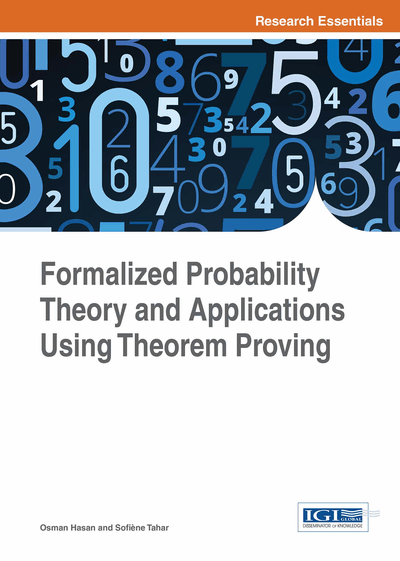# Reliability Theory

DOI: 10.4018/978-1-4666-8315-0.ch012
Available
\$37.50
No Current Special Offers

## Abstract

In this chapter, some basic concepts of reliability theory, namely cumulative distribution function, survival function and hazard function, and reliability block diagrams, are described and their higher-order-logic formalization is presented. Some of the important properties of these reliability concepts are formally verified using the HOL4 theorem prover to facilitate reasoning about reliability of engineering systems.
Chapter Preview
Top

The foremost foundation for reliability analysis of engineering systems (Bilintion & Allan, 1992) is lifetime distribution. Various lifetime distribution representations have been used in the literature depending upon the specific needs of a lifetime reliability analysis problem. For example, sometimes the probability of failure is of interest at a certain time (Survival function), whereas, in some other applications, such as in planning for serviceability and maintainability of a system, the total amount of risk associated with a system up to a given time (Cumulative Hazard function) may be required. Two other commonly used important reliability properties are the hazard function and the fractile function. The hazard function expresses the failure risk at a given time and the fractile function allows reasoning about the times of failure corresponding to a given probability of failure. The survival function ST (t) is defined as:ST (t) = 1 – FT (t)(12.1) where FT (t) is the cumulative distribution function of the random variable T. The Hazard function, hT (t), is defined as:

(12.2) and the cumulative hazard function, HT (t), is defined as:

(12.3)

Finally, the pth fractile tT (p) of a random variable T is defined as:

(12.4)

The main scope of this chapter is the formalization cumulative distribution function and survival function in higher-order-logic and the formal verification of some of their key properties. This formalization can then be utilized to formalize other lifetime distributions or reason about the reliability aspects of engineering systems within the sound core of a theorem prover (Hasan, Tahar, & Abbasi (2014); Abbasi, Hasan & Tahar (2010); Abbasi, Hasan, & Tahar, (2012); Abbasi, (2012); Abbasi, Hasan & Tahar, 2014)).

Top

## 12.2 Cumulative Distribution Function

In this section, we present the formal specification of the cumulative distribution function (CDF) andthe verification of CDF properties in the HOL4 theorem prover. The CDF and its properties have been an integral part of any reliability analysis as all the lifetime distributions are primarily based on CDF.

Mathematically, the CDF is defined as follows:

(12.5)

It follows from Equation (9.5) that the CDF can be formally specified in HOL4 by a higher-order-logic function that accepts a random variable as an argument and returns the probability of the event when the given random variable is less than or equal to the value of any given real number. A formalization of the CDF has already been given in Definition 5.6 and we repeat it here:

• Definition 12.1

`Ⱶ ∀ p X. CDF p X x = distribution p X { y | y ≤x }`

where X represents the random variable, p is the probability space and x is the real argument. Using this formal specification of the CDF, we can verify the classical CDF properties within the HOL4 theorem prover. The formal proofs for these properties not only ensure the correctness of Definition 9.1 but also play a vital role in proving various reliability properties associated with the lifetime distributions.

## Complete Chapter List

Search this Book:
Reset